# Frobenius Elements

Suppose that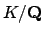is a finite Galois extension with group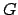and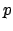is a prime such that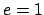(i.e., an unramified prime). Then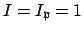for any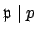, so the map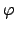of Theorem 14.1.5 is a canonical isomorphism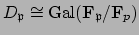. By Section 14.1.1, the group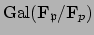is cyclic with canonical generator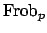. The corresponding to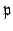is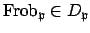. It is the unique element ofsuch that for all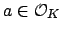we have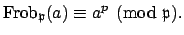(To see this argue as in the proof of Proposition 14.1.8.) Just as the primesand decomposition groups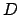are all conjugate, the Frobenius elements over a given prime are conjugate.

Proposition 14.2.1   For each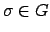, we have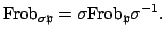In particular, the Frobenius elements lying over a given prime are all conjugate.

Proof. Fix. For anywe have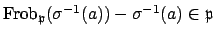. Multiply by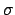we see that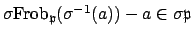, which proves the proposition.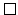Thus the conjugacy class of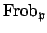inis a well defined function of. For example, ifis abelian, thendoes not depend on the choice oflying overand we obtain a well defined symbol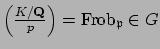called the . It extends to a map from the free abelian group on unramified primes to the group(the fractional ideals of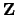). Class field theory (for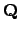) sets up a natural bijection between abelian Galois extensions ofand certain maps from certain subgroups of the group of fractional ideals for. We have just described one direction of this bijection, which associates to an abelian extension the Artin symbol (which induces a homomorphism). The Kronecker-Weber theorem asserts that the abelian extensions ofare exactly the subfields of the fields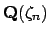, as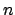varies over all positive integers. By Galois theory there is a correspondence between the subfields of(which has Galois group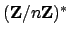) and the subgroups of. Giving an abelian extension ofis exactly the same as giving an integerand a subgroup of. Even more importantly, the reciprocity map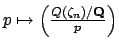is simply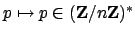. This is a nice generalization of quadratic reciprocity: for, the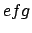for a primedepends in a simple way on nothing but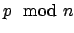.

William Stein 2004-05-06